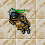## Sunday, August 1, 2021

### TibiaGoals Riddles E10R09

Comment down below!

1.3x3=9

Lovely Olka

2.3.3x3=9

Shizurie

4.By moving the upper left vertical arrow of the number 5 to the right side so the 5 forms a 3 instead. 3 x 3 = 9.

-Essiee

5.Hey! :D Moving the top-left arrow of the 5 to the top-right of it changes it into a 3, so the equation says 3 x 3 = 9.
Thanks a lot and have a wonderful start to your week! :) - Elyrea

6.Neo

By moving the top left vertical arrow of the 5 to the right, it would be a 3, making it 3x3=9

7.3 x 3 = 9

Pameliin

8.respuesta es: 3*3=9.... Zazy torturador sombrio

9.Move the top left arrow from the 5 to the right side to make a 3 making the equation 3x3=9

Xarkost Eilia

10.11.12.3x3 = 9
Vysterion

13.3x3=9; Lord Neganek

14.One option: Remove top left arrow from number '5'and putting it to top right making it number'3'. 3x3=9

Yeka

15.3x3 = 9

Skyelie Hasulhoff <3

16.17.3*3=9 .... Bebitha consentida

18.3*3=9 .... Bebitha consentida

19.Turning the 5 into 3
3 x 3 = 9

Char: Dyaniixz

20.moving the arrow on the number 5 to make it a number 3 so ecuation will be 3x3=9

character name: Pablo Reformed

21.moving the arrow on the number 5 to make it a number 3 so equation will be 3x3=9

character name: Pablo Reformed

22.3x3=9
Mian Stone'arrow

23.Move the top right arrow in the 9 to make it 15. 3 x 5 = 15

Toxic Hasulhoff

24.Move the top right arrow in the 9 to make it a 5 and place the arrow next to it making it a 15.
3 x 5 = 15

Toxic hasulhoff

25.3 x 3 = 9

Char: Marulinha Chatinha

26.Change 5 to 3, 3x3=9
Eternal hunger
Antica

27.Change 5 to 3, 3x3=9
Eternal hunger
Antica

28.Change 5 to 3, 3x3=9
Eternal hunger
Antica

29.30.Tamarizz - Solidera

By moving one arrow from the number 5, making it a 3 so it would be 3x3=9